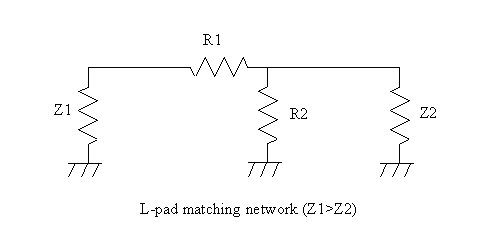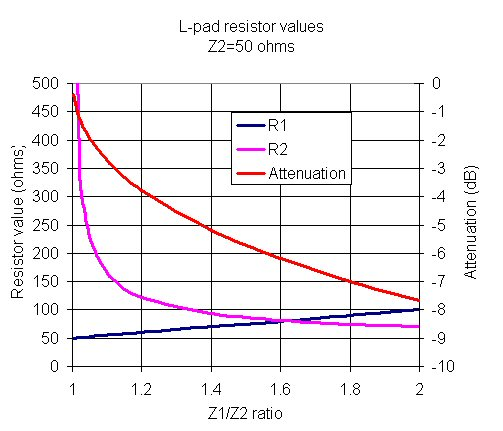L-pad attenuators are popular with audio geeks throughout the world, but they don't get much play in the microwave industry. Here we'll tell why that is.

L-pads can be used to perfectly match one impedance to another, but the solution is lossy. In microwave engineering, this is usually unacceptable, we'd rather match two impedances with near-zero loss by using reactive components, which limits the bandwidth of the solution. Note that for a given input and output impedance, only one solution exists for an L-pad where the input and output are to be perfectly matched; this is often called the "minimum loss matching pad".L-pads can be also be used as variable attenuators, if you allow one of the impedances to be mismatched, which is usually not a good idea for microwave circuitry because the effects of voltage standing waves (VSWR). Audio geeks do this all the time, they keep the generator side matched (so you don't blow up an expensive power amp), and to heck with the speaker side! At audio frequencies you don't have to worry about the effects of standing waves, unless your amplifier is in New York and your speaker is in California and they are hooked up by a lossless transmission line. Good one!

This configuration is often called the "minimum loss matching pad". It is used to match one real impedance to another real impedance, a typical application is to match 50 ohm stuff to 75 ohm stuff (we'll use that as an example below). Both source and load see matched impedances when this is done correctly.

The equations are simple to derive using Ohm's Law, or you can look them up in the ITT Handbook or other resources. For Z1>Z2,

R1=Z1xSQRT[1-(Z2/Z1)]

R2=Z2/SQRT[1-(Z2/Z1)]

Note: be sure to assign Z1 to the higher value of impedance and follow the convention of the figure as to which side the series resistor R1 goes on.

Updated September 2011! We've added some missing brackets in the Pout/Pin equation below, thanks to Quang from New Zealand. Thanks!

The attenuation (or insertion power lost) is defined as (Power out)/(Power in). When you derive this formula you have to be careful to include the effects of the disparate input and output impedances.

Pout/Pin=1/[SQRT(Z1/Z2)+SQRT((Z1/Z2)-1)]^2

(linear, not decibels!)

These calculations are in an Excel spreadsheet that is available in our download area, check it out!

Let's look at an example. Suppose you wanted to create the perfect match between a 50 ohm load (right side of figure) and a 75 ohm generator (left side of figure). Using the above equations,

R1=43.3

R2=86.6

Attenuation (dB)=-5.72 dB.

Note: if you wanted to match a 50 ohm generator to a 75 ohm load, the values of R1 and R2 are the same, but you need to turn the network around....

This begs the question... why would anyone want to throw away almost 75% of their available power, when the mismatch loss between the two impedances is only 0.2 dB? Resistive matching is only used where you don't particularly care about efficiency, but are concerned about achieving a good impedance match.

A classic solution to mismatched impedances at microwave frequencies is the quarterwave transformer which is lossless in the ideal case.

Let's look at a range of impedance mismatch from 50 ohms, for 50 to 100 ohms in the plot below. for matching 60 ohms to 50 (Z1/Z2=1.2), you will lose almost 4 dB (red line). For a 2:1 mismatch (100:50 ohms), you pay an attenuation penalty of 8 dB. So the phrase "minimum loss pad" is a cruel joke indeed!Author : Unknown Editor